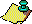Contents Next: Remark. Up: The Floating-Point Gauss Previous: The Floating-Point Gauss

The following theorem justifies the remarks of the previous section. The basic idea of its proof is that given some initial point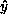the floating-point Gauss map also generates an initial point y whose continued fraction representation is exactly equal to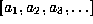, where the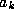are all (machine representable) integers. This initial point y has a G-orbit that is everywhere within a small multiple of u, the machine epsilon, of the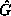-orbit of. The technique of the proof is of interest for more than just the Gauss map, because similar techniques can be used to prove that numerical simulations of orbits of some continuous systems are machine close to exact orbits of some nearby initial point (for a descriptive review of work by Yorke, Grebogi, and Hammel establishing similar results for continuous maps see ).

### Theorem 4

If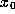,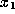,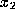,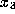,is the sequence of iterates of, and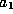,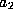,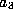,is the sequence of (machine representable) integers that arise in the process, then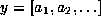has an orbit under G whose elements are close to,,in a sense to be made precise, and, in particular, y is close to.

### [Proof]Contents Next: Remark. Up: The Floating-Point Gauss Previous: The Floating-Point Gauss